# Liquid Conductivity Measuring conductivity in saline water solutions

• Slides: 22• Liquid Conductivity Measuring conductivity in saline water solutions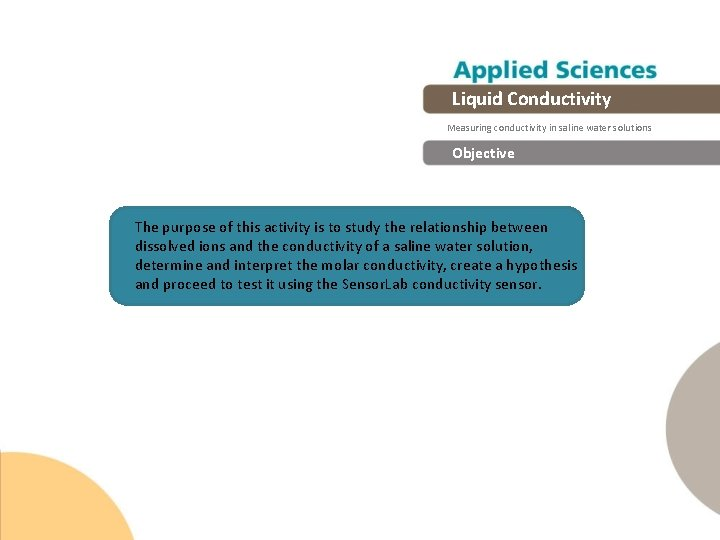Liquid Conductivity Measuring conductivity in saline water solutions Objective The purpose of this activity is to study the relationship between dissolved ions and the conductivity of a saline water solution, determine and interpret the molar conductivity, create a hypothesis and proceed to test it using the Sensor. Lab conductivity sensor.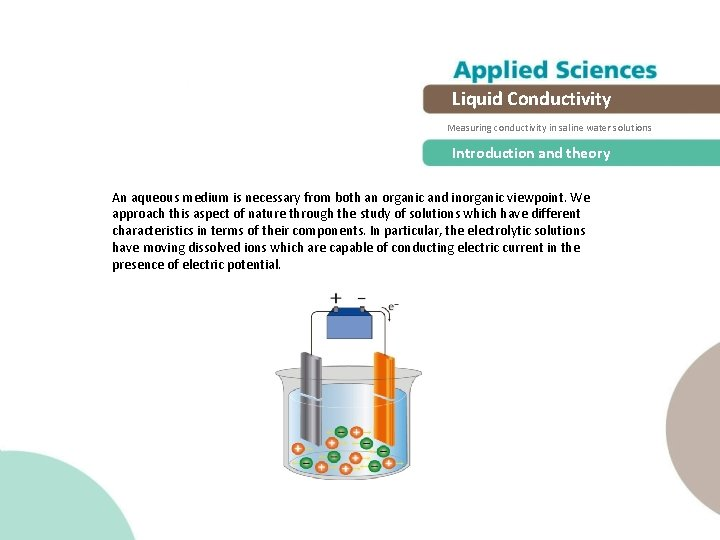Liquid Conductivity Measuring conductivity in saline water solutions Introduction and theory An aqueous medium is necessary from both an organic and inorganic viewpoint. We approach this aspect of nature through the study of solutions which have different characteristics in terms of their components. In particular, the electrolytic solutions have moving dissolved ions which are capable of conducting electric current in the presence of electric potential.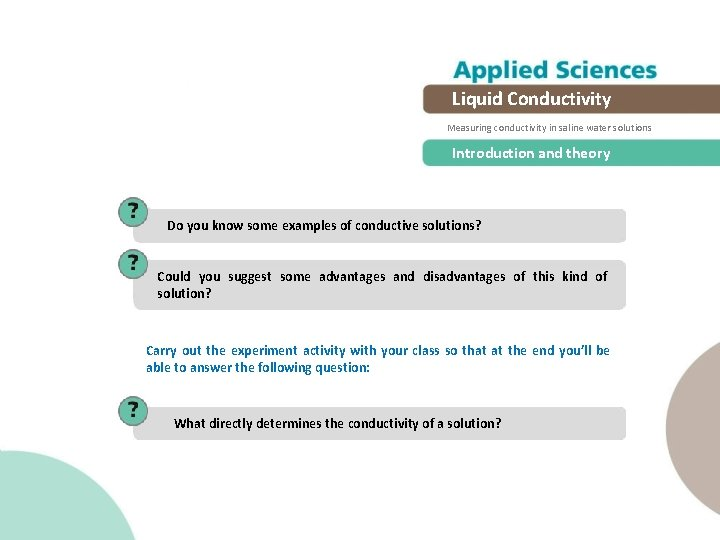Liquid Conductivity Measuring conductivity in saline water solutions Introduction and theory Do you know some examples of conductive solutions? Could you suggest some advantages and disadvantages of this kind of solution? Carry out the experiment activity with your class so that at the end you’ll be able to answer the following question: What directly determines the conductivity of a solution?Liquid Conductivity Measuring conductivity in saline water solutions Introduction and theory Theoretical In ionic solutions it is important to know how easily the electric current passes i. e. conductivity. The conductivity depends on the solute’s features, and on the concentration of dissolved ions which are responsible for the electric flux. Strong electrolytes are solutes that completely dissociate in a solution (HCl, Na. Cl, KOH) and show the general relation for electrolytes: cΛ=k Λ: Molar conductivity [m. S cm-1 M-1] k: Conductivity [m. S cm-1] c: Concentration [mol/L or M] The molar conductivity is the proportion between the solution conductivity and its concentration.Liquid Conductivity Measuring conductivity in saline water solutions Introduction and theory Now students are encouraged to raise a hypothesis which must be tested with an experiment. How are the solution concentration and conductivity related?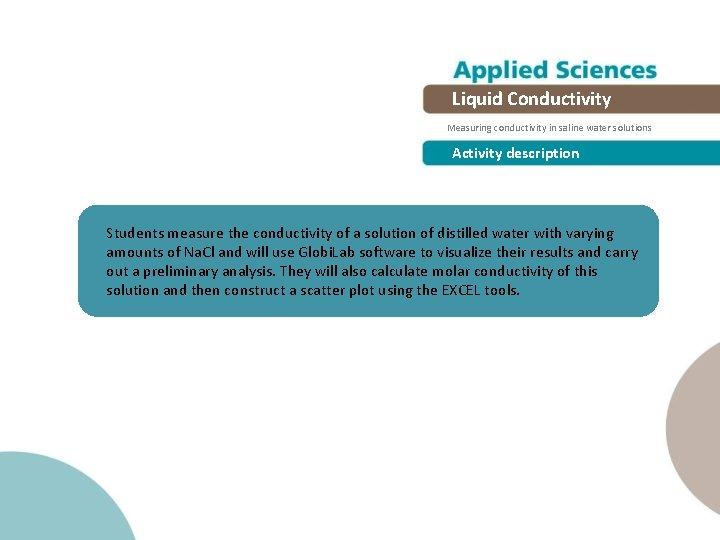Liquid Conductivity Measuring conductivity in saline water solutions Activity description Students measure the conductivity of a solution of distilled water with varying amounts of Na. Cl and will use Globi. Lab software to visualize their results and carry out a preliminary analysis. They will also calculate molar conductivity of this solution and then construct a scatter plot using the EXCEL tools.Liquid Conductivity Measuring conductivity in saline water solutions Resources and materials 1 2 3 4 5 6 Sensor. Lab Biochem Beaker (500 ml) Conductivity electrode Stirrer bar Distilled water Salt 4 3 2 1Liquid Conductivity Measuring conductivity in saline water solutions Using the Sensor. Lab configuration To collect measurements with the Sensor. Lab and conductivity electrode, the Sensor. Lab must be configured according to the following steps: 1 Open the Globi. Lab software and turn on the Sensor. Lab 2 Click on the Bluetooth icon in the bottom right corner of the Globi. Lab screen. Select the Sensor. Lab you are currently using. Once the Sensor. Lab has been recognized by the software, the icon will change from a grey to blue color. If you prefer a USB connection follow the previous instruction clicking on the USB icon. You will see the same color change when the Sensor. Lab is recognized.Liquid Conductivity Measuring conductivity in saline water solutions Using the Sensor. Lab 3 Click on to configure the Sensor. Lab. Select p. H in the “Logger Setup” window. Enter “Manual” for Rate.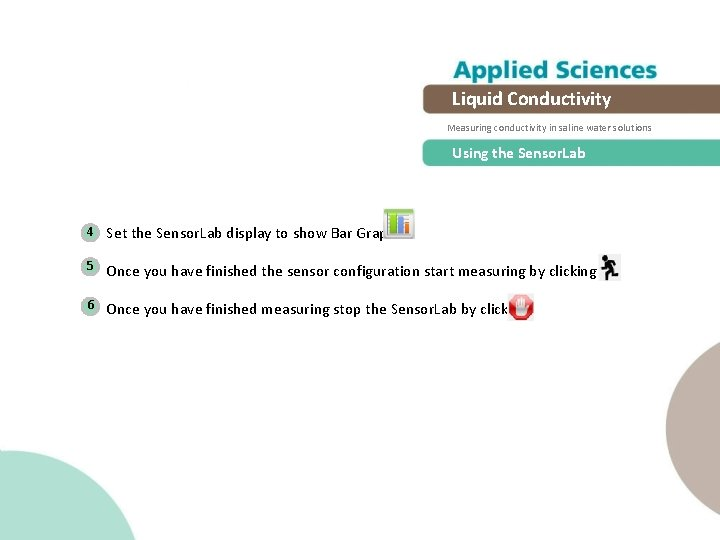Liquid Conductivity Measuring conductivity in saline water solutions Using the Sensor. Lab 4 Set the Sensor. Lab display to show Bar Graph 5 Once you have finished the sensor configuration start measuring by clicking 6 Once you have finished measuring stop the Sensor. Lab by clicking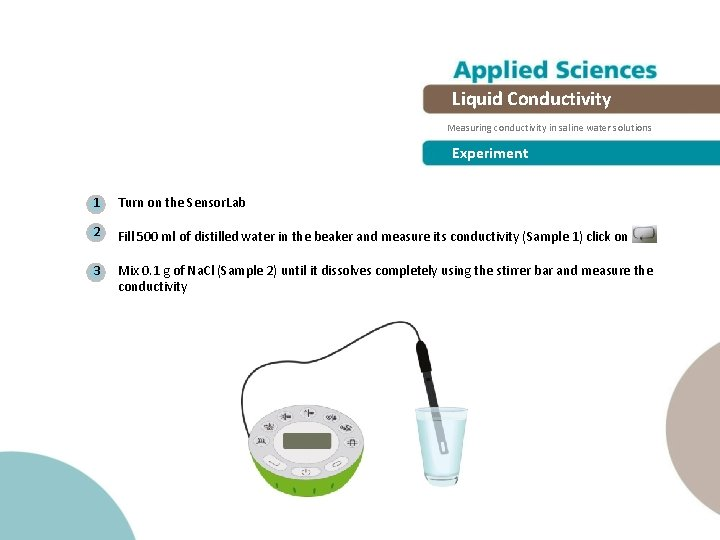Liquid Conductivity Measuring conductivity in saline water solutions Experiment 1 Turn on the Sensor. Lab 2 Fill 500 ml of distilled water in the beaker and measure its conductivity (Sample 1) click on 3 Mix 0. 1 g of Na. Cl (Sample 2) until it dissolves completely using the stirrer bar and measure the conductivity .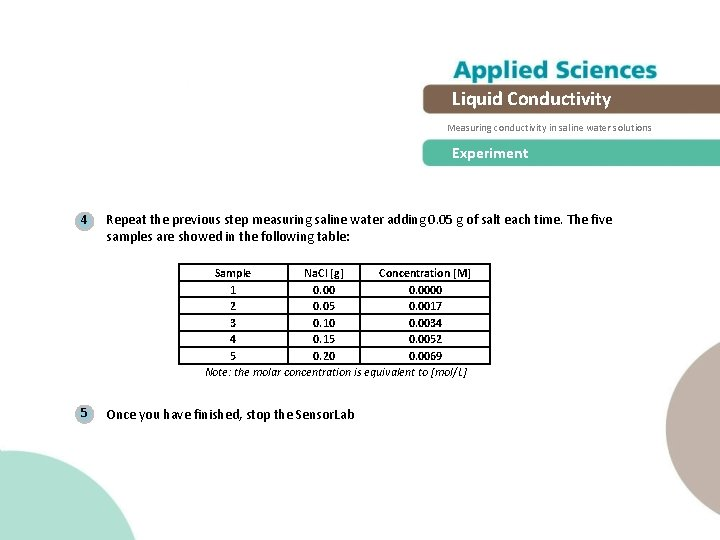Liquid Conductivity Measuring conductivity in saline water solutions Experiment 4 Repeat the previous step measuring saline water adding 0. 05 g of salt each time. The five samples are showed in the following table: Sample Na. Cl [g] Concentration [M] 1 0. 0000 2 0. 05 0. 0017 3 0. 10 0. 0034 4 0. 15 0. 0052 5 0. 20 0. 0069 Note: the molar concentration is equivalent to [mol/L] 5 Once you have finished, stop the Sensor. Lab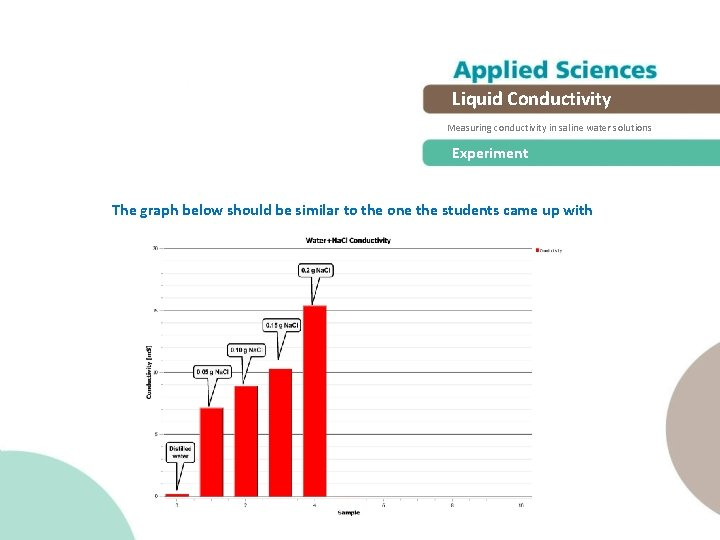Liquid Conductivity Measuring conductivity in saline water solutions Experiment The graph below should be similar to the one the students came up with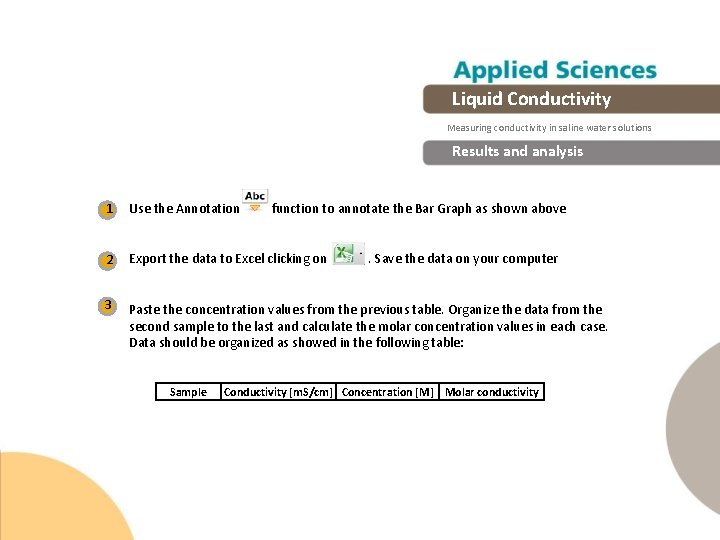Liquid Conductivity Measuring conductivity in saline water solutions Results and analysis 1 Use the Annotation 2 Export the data to Excel clicking on 3 Paste the concentration values from the previous table. Organize the data from the second sample to the last and calculate the molar concentration values in each case. Data should be organized as showed in the following table: Sample function to annotate the Bar Graph as shown above. Save the data on your computer Conductivity [m. S/cm] Concentration [M] Molar conductivity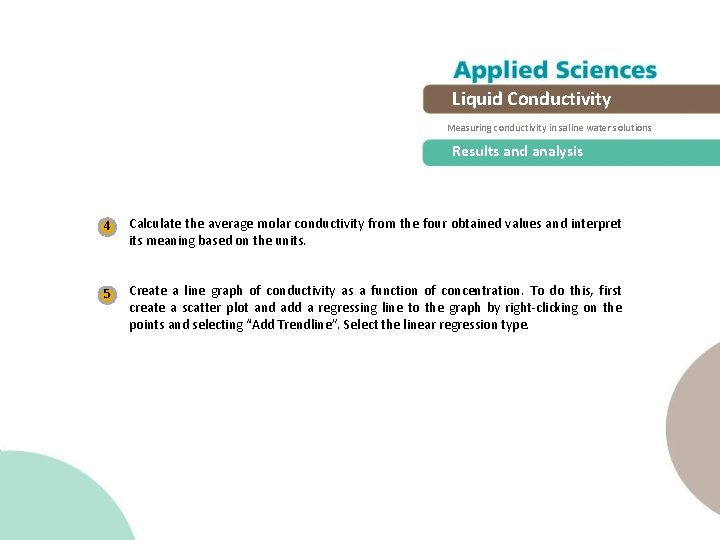Liquid Conductivity Measuring conductivity in saline water solutions Results and analysis 4 Calculate the average molar conductivity from the four obtained values and interpret its meaning based on the units. 5 Create a line graph of conductivity as a function of concentration. To do this, first create a scatter plot and add a regressing line to the graph by right-clicking on the points and selecting “Add Trendline”. Select the linear regression type.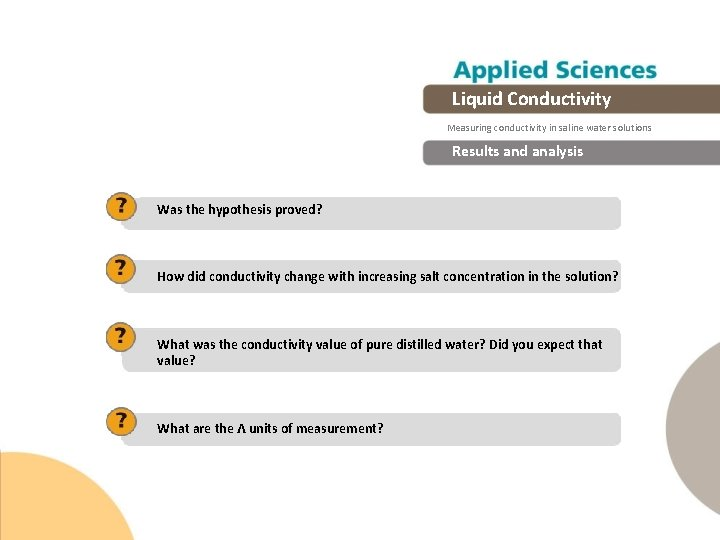Liquid Conductivity Measuring conductivity in saline water solutions Results and analysis Was the hypothesis proved? How did conductivity change with increasing salt concentration in the solution? What was the conductivity value of pure distilled water? Did you expect that value? What are the Ʌ units of measurement?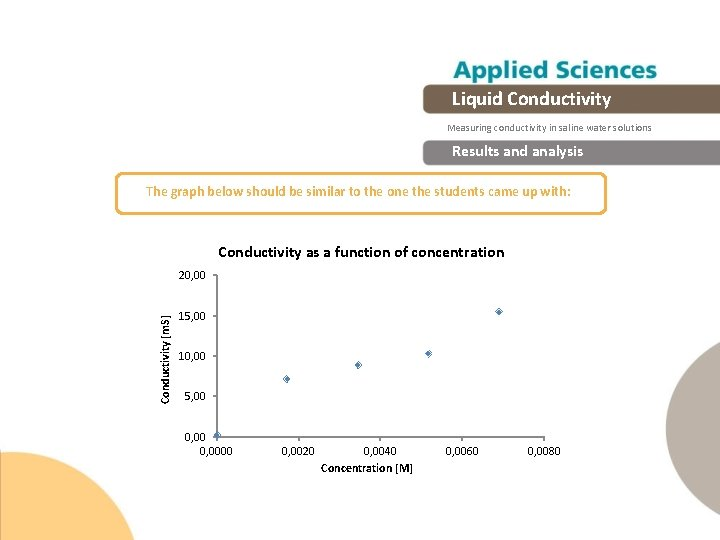Liquid Conductivity Measuring conductivity in saline water solutions Results and analysis The graph below should be similar to the one the students came up with: Conductivity as a function of concentration Conductivity [m. S] 20, 00 15, 00 10, 00 5, 00 0, 0000 0, 0020 0, 0040 Concentration [M] 0, 0060 0, 0080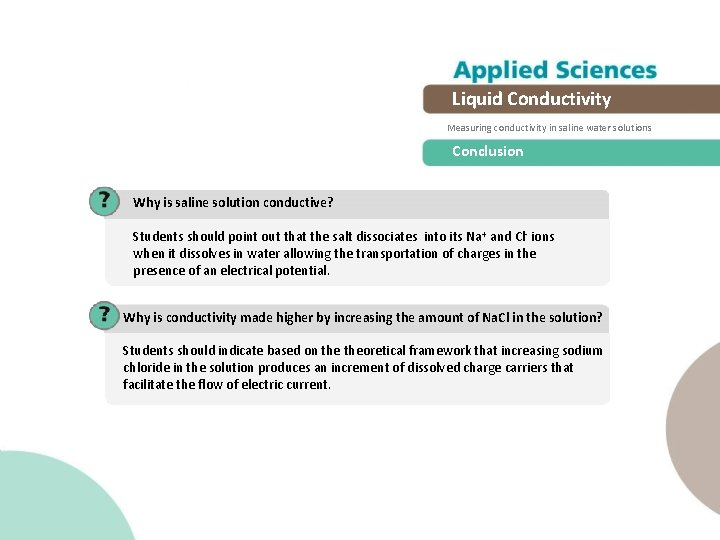Liquid Conductivity Measuring conductivity in saline water solutions Conclusion Why is saline solution conductive? Students should point out that the salt dissociates into its Na+ and Cl- ions when it dissolves in water allowing the transportation of charges in the presence of an electrical potential. Why is conductivity made higher by increasing the amount of Na. Cl in the solution? Students should indicate based on theoretical framework that increasing sodium chloride in the solution produces an increment of dissolved charge carriers that facilitate the flow of electric current.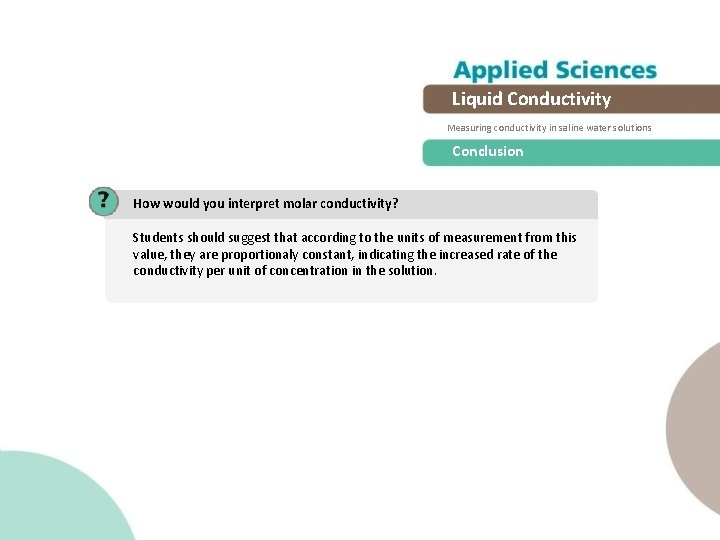Liquid Conductivity Measuring conductivity in saline water solutions Conclusion How would you interpret molar conductivity? Students should suggest that according to the units of measurement from this value, they are proportionaly constant, indicating the increased rate of the conductivity per unit of concentration in the solution.Liquid Conductivity Measuring conductivity in saline water solutions Activities for further application If you wanted to monitor the purity of water, would you use conductivity as an indicator? Explain. Students might point out that the purity of water is given by the concentration of different types of dissolved solutes. Many of them are separated into ions, such as sodium chloride, so that the conductivity reports give us an approximate value of the concentration of dissolved solutes. If you stir a volume of distilled water with your fingers, will the conductivity vary? Students should suggest that the excretion of salts through the skin produces a little conductivity change in distilled water.